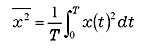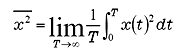FFT analyzer FAQWhat is Signal Power?The value before calculating the square root of the equations (1) or (2) as described in[What is Effective (RMS) value?]is called mean square value. In other words, it is expressed as follows. If periodic signal is x (t);(1)

and if non-periodic signal is x (t);(2)

This mean square value is called power of signal x (t). And the value excluding averaging in equation (1) or (2) is called energy of the signal x (t). For this reason, power of the signal x (t) could be called the time averaging value of the energy of the signal x (t).

When the physical unit of the signal x (t) is EU, the unit is EU2 for the power, EU2 s for the energy.

* Power or Energy of signal x (t) as is defined here is used in the signal processing field, is not always corresponding to the physical amount.

Revised:2009/11/16